# Sliding Fuzzy Controller for Energy Management of Residential Load by Multi-sources Power System Using Wind PV and Battery

Sliding Fuzzy Controller for Energy Management of Residential Load by Multi-sources Power System Using Wind PV and Battery

Electrical Engineering Department, University Tahar Moulay of Saida, BP 138 cité ENNASR 20000, Saida, Algeria

Laboratory of L2GEGI, Faculty of Applied Sciences, University of Tiaret, BP 78 Tiaret 14000, Algeria

Corresponding Author Email:
mostefa.koulali@univ-tiaret.dz
Page:
305-315
|
DOI:
https://doi.org/10.18280/jesa.530301
12 October 2019
|
Accepted:
24 January 2020
|
Published:
31 July 2020
| Citation

OPEN ACCESS

Abstract:

This work is interested in the study and analysis of the functioning of a hybrid energy production system made up of three energy sources: Wind energy, a photovoltaic generator, and a battery. The control of each part of the multi-source production chain generates the optimization of the functioning of the global system. The multi-level inverter is inserted in the chain of the hybrid system which can also improve the quality of the energy injected into the alternating load and consequently reduce the harmonic rate. The stability of the voltage across the DC bus capacitor plays a very important role in the healthy functioning of the production chain. For this purpose, an adjustment based on a sliding mode controller which ensures the adjustment of the DC voltage. Among the solutions deployed in order to obtain an excellent quality of energy to the network or to the AC load is the use of multi-level inverters. The major interest of this work is to extract the maximum power from the DC bus based on fuzzy logic control. The load requirements are often varied and to meet them, an algorithm for managing the energy flow between the different production sources is proposed. The implementation of the proposed system is carried out under the Matlab / Simulink environment, the results obtained validate the effectiveness of the technique and its feasibility in simulation and even in the case of practical implementation in real time.

Keywords:

battery storage system, multi-sources system, three level inverter, MPPT, sliding mode control, fuzzy logic control, photovoltaic system, wind turbine

1. Introduction

Electrical energy from renewable sources, particularly the wind and the sun, is considered an attractive production alternative in the electrical energy systems of the world today.

However, their use is limited by the variability of resources which may reduce their reliability.

To overcome this limit, the solution to be adopted is certainly the recourse and the combination of several sources, such as hybrid wind-photovoltaic systems with battery [1, 2].

These are complex systems combining different renewable sources. They compensate for the intermittent nature of renewable sources and offer a high overall energy yield and the possibility of autonomy. Hybrid energy systems can be connected to electricity grids or installed in isolated sites.

Research and development efforts in solar, wind, and other renewable energy technologies are necessary to continue improving their performance. Among the most important factors to consider, we can cite the maximum power point tracking (MPPT), which presents an essential factor of system performances .

In the literature, different controlling types of both renewable energy sources and power electronic converters can be seen . In some research, control techniques such as fuzzy logic control, proportional integral (PI)-derivative control, and fuzzy neural networks are frequently used. The used chopper and inverter are controlled by these techniques.

The insertion of energy storage systems (ESS) in the production chain by renewable sources is the concern of researchers, the constraints encountered lie in the minimization of harmonic rates of harmonics, the stability of these systems, the flexibility of injection of this energy to the grid and the quality of energy transmitted all while ensuring the desired performance.

Several studies have demonstrated power smoothing in order to solve the problem related to energy quality , the battery energy storage system (BESS) is the best solution which makes it possible to obtain an energy balance between wind and photovoltaic renewable energy sources on the one hand and the electrical network and alternative load on the other. Fuzzy Logic Control (FLC) is introduced in order to properly control the three-level inverter control connected to the AC load, this control technique allows controlling the RMS value of the load voltage.

This paper investigates the design of a renewable energy production system associated with a storage system based on accumulator batteries in order to supply an alternative charge. Control algorithms using fuzzy logic and sliding mode are presented in order to optimize the functioning of the system, in addition an energy management between the different parts is taken into account in this work is able to adapt to a changing configuration. The research carried out presents a standard simulation model which ensures the control of the energy flow and the results of the simulation obtained clearly show the reliability and the feasibility of the proposed control method.

## 1.png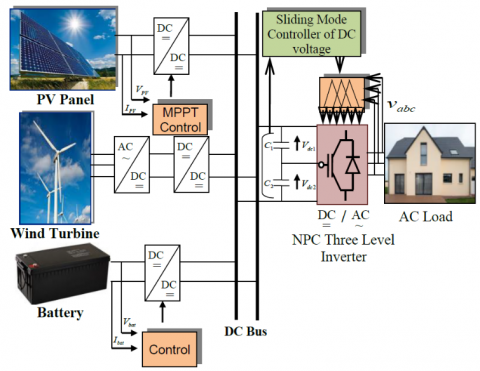Figure 1. Schematic of the PV-wind block diagram associated with the battery

2. Description of the Physical Elements Studies

Figure 1 shows the combination of solar wind sources and the battery storage system driven by the proposed fuzzy logic controller.

2.1 PV system modeling

A Photovoltaic solar cell is a large area PN electronic diode which, exposed to light (photons), generates an electrical voltage (volts), an ideal photovoltaic cell as shown in Figure 2 can be described in a simple way as an ideal source of current which produces a current proportional to the incident light power, in parallel with a diode . In addition to the model ideal, the real model takes into account the resistive properties of the cell which are modeled by a series resistance RS but also leakage currents modeled by a shunt resistor Rsh.

$I_{P V}=I_{p h}-I_{0}\left(\exp \left(\frac{q\left(V_{P V}+R_{s \bmod } I_{P V}\right)}{A K T}\right)-1\right)$       (1)

## 2.pngFigure 2. Scheme of PV cell

2.2 MPPT technique control for boost converter

The voltage delivered by the solar panels must reach a nominal value at the output facing the load. For this purpose, a DC / DC boost converter must be inserted to increase this voltage.

Figure 3 shows a solar panel connected to a boost converter which includes a DC input voltage Vin, an inductor L, a switch S, a diode D1, a capacitor C for the filter and a load resistor R.

## 3.pngFigure 3. PV diagram with the boost converter and its MPPT control

2.3 Operating principle of the PV boost assembly

When the switch S is on, the boost inductor stores the energy supplied by the input voltage source, at the same time the charged capacitor maintains the constant continuous charge current.

When switch S is off, the inductance voltage and the stored input voltage will appear at the load terminals are maximum.

When switch S is off, the stored inductance voltage and the input voltage will appear at the charging terminals are maximum. However, the charging voltage depends on the duty cycle D and on the state of the switch S to on or off.

Improving the efficiency of the solar panel is ensured by the MPPT technique. This algorithm is based on the maximum power extraction theorem. Maximum power is obtained by adapting the impedance of the source and making it equal to that of the load.

The duty cycle of the DC / DC converter is modified by extracting the maximum power from the solar cell and this by modifying and adapting the impedance.

2.4 Wind energy conversion system

2.4.1 Wind turbine modeling

The wind rotor is an energy conversion system that transforms energy kinetics of moving air masses in mechanical energy available on the shaft.

To capture the kinetic energy of moving air masses, the blades of the wind rotor present obliquely to the wind with an angle called the setting angle, p, causing the rotation by a screw or corkscrew effect. The maximum power that can be recovered is described by the Betz equation [5, 6]:

$P_{m e c}=\frac{1}{2} \rho A C_{P} V_{W}^{3}=C_{p} P_{W}$           (2)

where, $\rho$ is the air density [kg/m3]; A is the rotor swept area [m2]; CP is the power coefficient; Vw is the wind speed upstream of the rotor [m/s]; PW is the power extracted from wind [w].

The ratio $\lambda$, called the tip speed ratio, is give:

$\lambda=\frac{\omega_{V} R}{V_{W}}$         (3)

where, $\omega_{W}$ is the angular velocity of rotor [rad/s]; R is the rotor radius.

The power coefficient CP can be written as :

$C_{p}(\lambda, \beta)=0.5\left(116 \frac{1}{\lambda_{i}}-0.4 \beta-5\right) e^{-\frac{21}{\lambda_{i}}}$                   (4)

with: $\frac{1}{\lambda_{i}}=\frac{1}{\lambda+0.08 \beta}-\frac{0.035}{1+\beta^{3}}$

where, is the pitch angle.

The torque wind can expressed as:

$T_{W}=T_{m e c}=\frac{1}{2} \frac{C_{P} \rho R A V_{W}^{2}}{\lambda}$                 (5)

It should be noted that the mechanical torque transmitted to the generator is the same as the aerodynamics torque, since there is no gearbox.

The Figure 4 shows the model of the wind turbine.

## 4.pngFigure 4. Wind turbine model

2.4.2 Mathematic modeling of PMSG

The state model of the PMSG can be written as follows :

$\left\{\begin{array}{l}v_{s d}=R_{s} i_{s d}+L_{d} \frac{d i_{s d}}{d t}-\omega_{e} L_{q} i_{s q} \\ v_{s q}=R_{s} i_{s q}+L_{q} \frac{d i_{s q}}{d t}+\omega_{e}\left(L_{d} i_{s d}+\psi_{f}\right)\end{array}\right.$                               (6)

where, d, q are the physical quantities that have been transformed into the d-q synchronous rotating reference frame; RS is rotor resistance $|\Omega|$; Ld, Lq re the inductance of the generator on the d and q axis [H]; $\psi_{f}$ is the permanent magnetic flux [Wb]; we s the electrical rotating speed [rad/s] of the generator defined by:

$\omega_{e}=p \omega_{r}$       (7)

where, p is the number of pole pairs of the generator; wr is the mechanical rotational speed of the generator.

The electromagnetic torque is written as follows:

$T_{e m}=\frac{p}{\omega}\left(i_{q} e_{q}\right)=p \psi_{f} i_{q}$                (8)

The mechanical equation for the PMSG is expressed as:

$\frac{J d \omega_{r}}{d t}=T_{e m}-T_{l}-f \omega_{r}$           (9)

where, Tl is the load torque, J and f are the motor inertia and viscous friction coefficient respectively.

3. Control Strategy Applied

After having developed the modeling of the elements of the production chain, the following section is interested in the development of the control strategies proposed in this study.

3.1 Battery modeling and control

3.1.1 Battery modeling

The reliability and efficiency of the electricity distribution system improved by energy storage devices. Among the various energy storage devices, the battery is the most commonly used storage device for storing electrical energy. Recently, there are many models of batteries developed in references .

The model used in this work is represented by the equivalent circuit which contains a voltage source which is the open circuit voltage V, in series with an internal resistance R. Thus, the output voltage of the battery is:

$V_{b a t}=V-R I_{b a t}$           (10)

where, Vbat: is the voltage battery [V]; Ibat is the current battery [A].

The voltage and current of the battery strongly depends on the SOC, the temperature and the variations of the internal resistance.

The Figure 5 display the electric battery model.

## 5.pngFigure 5. The typical battery model

The simple battery model chosen in this study is considered to be sufficiently precise and reliable to assess the objectives, in particular energy management.

The state of charge (SOC) of the battery is characterized by the charging and discharging process as a function of time, variations in the sources of production and the variation in the charge. The SOC is described by equation :

$\operatorname{SOC}(t)=\left\{\begin{array}{c}\operatorname{SOC}(t-\Delta t)+P_{\text {bat}} \cdot \frac{\eta_{c h}}{C_{n} \cdot V_{d c}} \cdot \Delta t \\ \operatorname{SOC}(t-\Delta t)+P_{\text {bat}} \cdot \frac{1}{\eta_{d i s} \cdot C_{n} \cdot V_{d c}} \cdot \Delta t\end{array}\right.$                (11)

where, Δt is the time step; Pbat is the battery power; Cn is the nominal capacity of the battery; $\eta_{c h}$ and $\eta_{\text {dis}}$ are respectively the battery efficiencies during charging and discharging phase; Vdc is the nominal DC bus voltage.

At any time Δt, the SOC must check the following condition:

$S O C_{\min } \leq S O C(t) \leq S O C_{\max }$           (12)

where, SOCmin and SOCmax are respectively the maximum and minimum allowable storage capacities.

3.1.2 Battery control strategy

In order to ensure the required power, it is necessary to control the current produced by the battery. Let us not forget to take into account the constraints relating to the charge and discharge current limits and the maximum SOC limits.

Figure 6 shows the storage system connected to the electrical grid through a bidirectional buck boost converter with its control.

The storage system chosen has three operating modes, namely: Charging modes, discharge modes and floating modes depending on the energy characteristics. They are managed according to the DC bus voltage at the BESS coupling point.

However, the battery must ensure the DC bus voltage necessary for transferring power to the grid regardless of the operating mode presented.

During the charging mode, the switch S2 is activated and the converter functions as a boost circuit; otherwise, in the discharge mode, the switch S1 is activated and the converter operates as a buck circuit.

The switch S1 is closed when the voltage on the intermediate circuit is lower than the voltage reference. Alternately, the switch S2 is closed when the voltage on the intermediate circuit is higher than the voltage reference.

## 6.pngFigure 6. The battery and bidirectional buck-boost converter control strategy

The PV-battery system response to transient variations is characterized by an inherent time constant. In such cases, capacitors along the DC grid can act as virtual inertia to supply the shortfall or absorb the surplus of energy [11-13].

The equation below expresses the power of the DC bus link:

$V_{d c} i_{d c}=P_{P V}+P_{W}+P_{b a t}-P_{\text {load}}$            (13)

where, PPV: is the photovoltaic power [W]; PW: is the wind power [W]; Pbat is the battery power [W]; Pload is the load power [W].

By neglecting the losses in the various elements of the conversion chain, namely the power converters, the battery, the filter inductors and the transformer as well as the harmonics due to the switching switches, the expression of the active power of the production written in the form :

$V_{d c} i_{d c}=C V_{d c} \frac{d V_{d c}}{d t}=P_{P V}+P_{W}+P_{b a t}-P_{l o a d}$            (14)

where, C is inverter capacitor [mF]; idc is the DC bus current [A].

The battery plays the role of an intermediate element between the sources of energy production and the load. It is always maintained at a constant voltage whatever the constraints and the variations of load.

The battery power controls the capacitor voltage when the powers injected by the two back-to-back voltage source converters (VSC) are assumed to be constant at a well-defined instant.

3.2 Three-level inverter modeling

Three-level inverter has the advantages of having low harmonic distortion, low switching frequency, and lower common mode voltages, near sinusoidal output voltage, less requirement of filtering and above all it reduces the danger of motor failure due to high frequency switching dv/dt . Figure 7 shows the topology of a three level NPC inverter.

The three level inverter has a total of 27 switching states 33.

When the upper switches Sak1,Sak2 are in the on state, that corresponds to the state ‘1’. When the lower switches Sak3,Sak4 are on, that corresponds to state ‘-1’. When the auxiliary switches Sak2,Sak3 are on, that results in state ‘0’ .

The functions Fb km of connection are given by:

$\left\{\begin{array}{l}F_{k 1}^{b}=F_{k 1} \cdot \mathrm{F}_{k 2} \\ F_{k 0}^{b}=F_{k 3} \cdot \mathrm{F}_{k 4}\end{array}\right.$            (15)

where, m=1: the upper half arm and m=0: the lower half arm.

The phase voltage VAO, VBO, VCO can be written as:

$\left\{\begin{array}{l}V_{A O}=F_{11}^{b} V_{c 1}-F_{10}^{b} V_{c 2} \\ V_{B O}=F_{21}^{b} V_{c 1}-F_{20}^{b} V_{c 2} \\ V_{C O}=F_{31}^{b} V_{c 1}-F_{30}^{b} V_{c 2}\end{array}\right.$              (16)

Simple output voltages are written as:

$\left[\begin{array}{c}V_{A} \\ V_{B} \\ V_{C}\end{array}\right]=\frac{1}{3}\left[\begin{array}{ccc}2 & -1 & -1 \\ -1 & 2 & -1 \\ -1 & -1 & 2\end{array}\right]\left\{\left[\begin{array}{c}F_{11}^{b} \\ F_{21}^{b} \\ F_{31}^{b}\end{array}\right] V_{c 1}-\left[\begin{array}{c}F_{10}^{b} \\ F_{20}^{b} \\ F_{30}^{b}\end{array}\right] V_{c 2}\right\}$               (17)

## 7.pngFigure 7. Power circuit of a 3-level inverter

3.3 Sliding mode controller of DC voltage

The sliding mode technique is one of the most widely used controls in industrial systems which are very complex. The advantage of the technique in question is the robustness, the reliability and the possibility of implementation.

The sliding mode controller is therefore adopted in first time to control the voltage of the DC bus of the inverter. As Figure 8 shows the DC voltage and current that are generated by the two sources, while the DC current reference is generated by the controller based on the sliding mode proposed in this work to control the DC bus voltage. However, the battery current is controlled to optimize the degradation of the battery and the SC current is controlled to compensate for the power demand of the load and also to stabilize the DC bus voltage at a constant value.

The current flowing in the capacitor is given by the equation:

$i_{c}=C \frac{d V_{d c}}{d t}$       (18)

With: $i_{c}= i_{i n p u t}- i_{d c}$

We obtain the first derivative of DC Voltage by a state formula shows as:

$\dot{v}_{d c}=0 v_{d c}+\frac{1}{C} i_{c}=a v_{d c}+b i_{c}$             (19)

The error between the reference DC voltage and that measured is chosen to be the sliding variable s (the surface s can be chosen as s=e)

$s=e=v_{d c}^{*}-v_{d c}$        (20)

The condition $e \dot{e}<0$ should be satisfied in order to ensure the existence of sliding-mode surface and to ensure too vdc converges to $V_{d c}^{*}$.

The derivate error can be expressed as follows:

$\dot{e}=\dot{v}_{d c}^{*}-\dot{v}_{d c}=-K \operatorname{sign}(e)$                   (21)

Which give:

$\dot{v}_{d c}^{*}=\frac{1}{C} i_{c}=-K \operatorname{sign}(e)$          (22)

Finally, we obtain:

$i_{d c}=i_{\text {input}}-C\left[\dot{v}_{d c}^{*}+K \operatorname{sign}(e)\right]$            (23)

where, K is the tuning gain.

The system control loop is show in Figure 8:

## 8.pngFigure 8. Structure of SMC of DC voltage

3.4 Fuzzy Logic Control (FLC) based three level inverter design

Fuzzy logic provides a sturdy framework for achieving robust and simple solutions amid different approaches of Intelligent computation.

The overall topology of the FLC is shown in Figure 9. This model contains a fuzzifier, a knowledge base, an inference engine and a defuzzifier [17, 18].

The performance of the system is degraded due to the three-level inverter which delivers a distortion of the voltage and the current coming out of the load.

## 9.pngFigure 9. Structure of FLC

## 10.png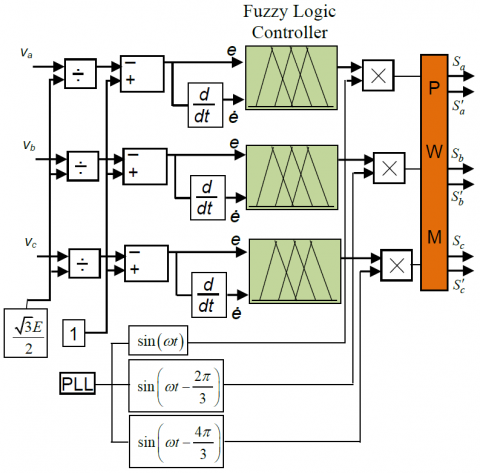Figure 10. Proposed FLC of three-phase 3-level inverter

## 11.png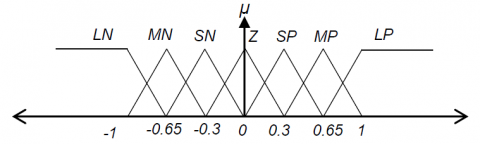Figure 11. Membership function for input variables

However, to solve these problems, an FLC fuzzy logic command is used to optimize the operation of the entire system.

In the FLC, there is no need the mathematical model of system and the choosing of rules depends on the knowledge experts [19, 20]. In this method, the switching signal of three level inverters is determined based on the error between the reference of magnitude voltage load and actual voltage output . In this case, the FLC has two inputs, the first input is error and the second input are change of error with one output the magnitude of RMS voltage load as shown in Figure 10.

The reference voltage of the NPC inverter is set and adjusted according to the changes in the input of the fuzzy controller. The number of rules can be defined according to an optimization policy. A 49 rules constituting the rule base, which are based on the seven membership functions of the input variables.

The rule base for the FLC is shown in Table 1.

Figure 11 shown the basic fuzzy sets of membership functions for the variables. The fuzzy variables are expressed by linguistic variables small negative (SN), medium negative (MN), large negative (LN), zero (Z), large positive (LP), medium positive (MP), small positive (SP), for all variables.

The knowledge base consists of a data base with the necessary linguistic definitions and control rule set. The rule set of knowledge base consists of some fuzzy rules that define the relations between inputs and outputs.

Table 1. Fuzzy logic check rules base

 e de LN MN SN Z SP MP LP LN LP LP LP MP MP SP Z MN LP MP MP MP SP Z SN SN LP MP SP SP Z SN MN Z MP MP SP Z SN MN MN SP MP SP Z SN SN MN LN MP SP Z SN MN MN MN LN LP Z SN MN MN LN LN LN

where,

LP: Large Positive,

MP: Medium Positive,

SP: Small Positive,

Z: Zero,

SN: Small Negative,

MN: Medium Negative,

LN: Large Negative.

3.5 Power management algorithm applied

The brain of this paper is the algorithm for managing renewable energy produced and storage in the battery to ensure optimal electrical consumption with maximum satisfaction.

The power levels of the storage system and the flows of renewable energy produced are the conditions for the smooth running of the energy management algorithm. Figure 12 shows the diagram of the energy management system proposed in this study.

## 12.png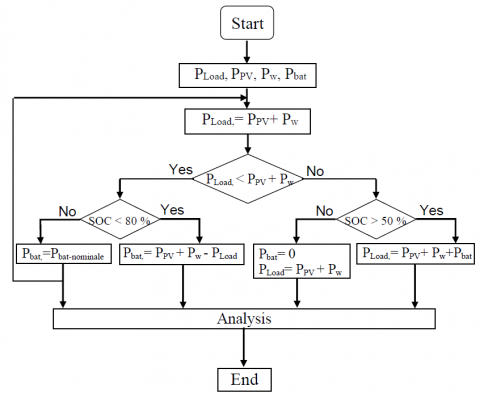Figure 12. Optimal energy management flowchart

The algorithm used takes into account the following sequence:

First point: define the different powers available in this system,

Second point: If the requested power is less than that delivered, in this case, the excess power will be stored in the battery,

Third point: If the power requested is greater than that generated, we distinguish the following two cases:

a) The storage battery system is activated if the SOC state of charge of battery is less than 50%;

b) In the opposite case, that is to say the state of charge of battery SOC is greater than 80%, then the battery ceases to function (switched off), which forces load shedding.

4. Simulation Results and Interpretations

The validation of the control method must be ensured by high performance obtained using Matlab / Simulink.

Tables 2, 3 and 4 give the numerical values of the parameters of the wind turbine and PV, the battery and the AC load, the DC converter and the inverter respectively.

Table 2. Wind turbine and PV parameters

 Components Rating values Peak Power 200 W Peak Voltage 660 V Peak current 7.52 A Open Circuit Voltage 33.2 V Short Circuit Current 8.36 A Rated power (Wind) 8.5 kW Type of Generator PMSG Rated Speed 1800 Number of poles 10

Table 3. AC load and battery parameters

 Components Rating values Load 1, 2, 3 (R) 7 kW, 7 kW, 3 kW Battery type Nickel Metal hydride Nominal voltage 300 V Capacity rating 6.5 AH

Table 4. Three level NPC inverter and DC/DC bi-converter

 Components Rating values C1=C2 2.2 mF Dc link Voltage 640 V Frequency 50 Hz Converter Inductor 5 mH DC link Voltage 640 V Converter Capacitor 2.2 mF

An input for the variable solar irradiation of the photovoltaic generator is proposed G = [600, 1000, 800, 1000] W/m2 at times t = [0-2, 2-3.5, 3.5-5.4, 5.4 -10] s respectively at a temperature T = 293 K.

Seven possible operating modes proposed which depends on the variable load.

Figures 12 to 18 show the performance of the system for different scenarios, the characteristics and performance of the PV generator are shown in Figure 13. The characteristics of the battery and the wind turbine (power, current, voltage and SOC) are displayed in Figures 14 and 15 respectively.

The storage device SOC is shown in Figure 14. The SOC is loaded at 60% between instants [0-3] s and the system are at full load. When the total power produced by the PV and the wind is greater than the requested power, the storage device is switched off in this case, this scenario is presented in Figure 16. When the system is in critical condition, the SOC continues to decrease, which allows the supervisor to react correctly to this situation and therefore cuts the load with the lowest priority in order to save the balance of the overall system.

When faced with the situation of lack of electricity, it is essential to introduce the screening technique, therefore, all storage devices would be disconnected.

Otherwise, the storage system charging mode is authorized. These results show the efficiency of energy management in addition to the control techniques used for this hybrid system.

## 13.png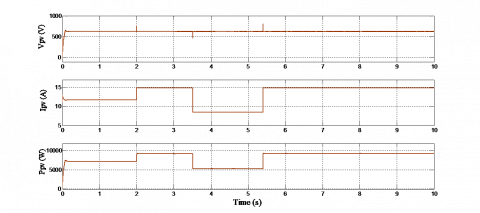Figure 13. PV generator output performances: voltage, current and power under varying irradiation lev

## 14.pngFigure 14. Battery performances: Voltage, current, power and Global State of Charge (SOC) of battery

## 15.pngFigure 15. Wind generator output performances: Voltage, current and power

Figure 16 shows the power distribution (load, PV, wind and battery).

Initially, between t=0 s and t=0.2 s, the load is varied from 0 kW to 7 kW. The grid can supply the power to the load and the PV charges the battery storage system. At t=2 second, the grid is disconnected and the load which is always set at 7 kW is supplied simultaneously by the PV and the battery. We notice at t=2s, the battery begins to discharge, the PV reacts to make the compensation. Then, the load is increased from 7 kW to 14 kW between t=3s and 4s. As a result shown, with this variation, the PV remains constant. However, it is the battery which passes to the compensation which corresponds to satisfy the new power demand.

## 16.pngFigure 16. Energy produced according to the variation of the AC load

One important performance measure of a hybrid system is the voltage of DC-link between the sources side the grid-side 3 level converter, as shown is Figure 17. The DC bus voltage, responses with the applied controller remain outstandingly insensible to the variation of the irradiation or wind speed with smaller ripple.

As it can be seen that with changing power, the DC link voltage Vdc is well kept constant at the specified value (640 V) which constitutes an important advantage and proves the effectiveness of the proposed schema. It has allowed us to equalize the different input DC link voltages of the multilevel inverter. Then, the input voltages are practically equal by pairs.

The currents and the voltages at the level of the load are purely sinusoidal. This result shows the efficiency of the proposed control at the inverter and a better quality of energy supplied (Figure 18 (a) and (b)).

## 17.pngFigure 17. DC bus voltage

## 18a.png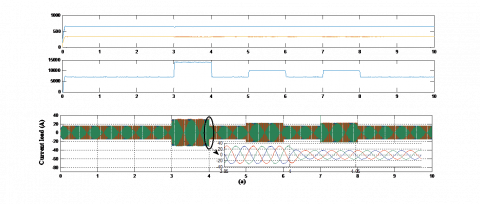## 18b.png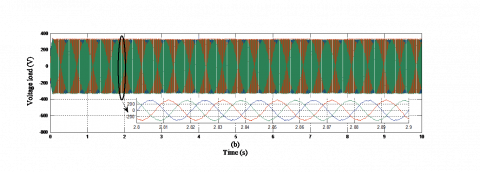Figure 18. Output of inverter and its zoom: (a) current, (b) voltage

It’s noticed that the currents and the voltage obtained by inverter conversion using the proposed control method presented a good THD 3.68% in Figure 19. Given the results obtained, it can be seen that the quality of energy is good and in international standards that does not exceed 5.5%.

The proposed algorithm advantageously reduces the harmonics and the ripple of the load currents and voltages.

We applied two types of controls, these are fuzzy and sliding mode regulators. The first consists in supplying energy to the load to ensure the stability between demand and generation while the second consists in ensuring the stability of the voltage against the voltage drop of the DC bus. From these results, it can be concluded that the energy management algorithm is capable of obtaining good control and a good balance between the power required by the load and the generation of multi-source system under the conditions of simulated operation.

## 19a.png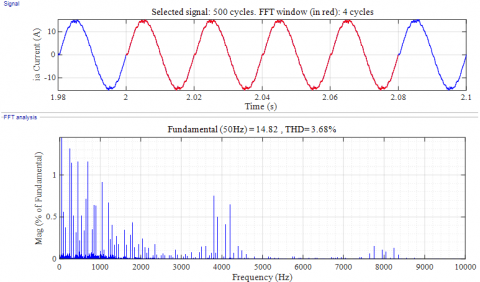(a)

## 19b.png(b)

Figure 19. Spectrum analysis of inverter output: (a) current load, (b) voltage load

5. Conclusions

This paper presents a fuzzy based optimized and sliding mode control for DC voltage and energy management system of a standalone hybrid power system, consisting of wind turbine and PV as primary renewable energy sources and battery as back up energy storage systems.

The energy delivered to the DC bus by the PV generator and the wind turbine is converted by a three-level inverter. The circuit breaker is used to connect and disconnect a residual load according to the energy management studied.

A control performances of a DC bus voltage is ensured by a sliding mode regulator to keep the system stable in the event of under transient condition (like sudden load removal). The SOC of the battery is optimized and monitored by a controller to meet the needs of the load, the PV radiation allocates the net power plus the power of the sources. The results of the simulation clearly show that the performance of the proposed system is satisfactory in different source and load variation cases and can be easily implemented with the Dspace or DSP controller board platform.

In addition, supplying electrical power by ensuring the continuity of the service to the load is the main ultimate objective achieved.

As perspectives, the study can be extended to a system which contains a diesel generator, a fuel cell and the supercapacitors including advanced control strategies.

Nomenclature
 BESS Battery Energy Storage System PMSG Permanent Magnet Synchronous Generator RMS Root-Mean-Squared THD Total Harmonic Distortion SOC State of charge of the battery Ibat Battery current [A] Id, Iq direct and quadratic stator currents [A] idc DC-link current [A] Iph Photocurrent of a solar PV cell generated due to solar irradiation [A] IPV Photovoltaic current [A] I0 Diode’s Reverse saturation current [A] A Curve fitting or diode ideality constant Rs Series resistance [Ω] T Absolute temperature [°K] K Boltzmann constant [1.3806503*10-23J/K] q Charge of electrons [C] PPV Photovoltaic system output power [W] Q Reactive power [VAR] RC Resistance of each stator phase [Ω] Tem Electromagnetic Torque [Nm] Vbat Battery voltage [V] Vd, Vq direct and quadratic stator voltage [V] Vdc DC link voltage [V] VPV Photovoltaic system output voltage [V] VSC Voltage Source Converters ψf Magnet flux [Wb] ωr Rotor speed [rad/s]
References

 Oskouei, A.B., Banaei, M.R., Sabahi, M. (2016). Hybrid PV/wind system with quinary asymmetric inverter without increasing DC-link number. Ain Shams Engineering Journal, 7(2): 579-592. https://doi.org/10.1016/j.asej.2015.06.008

 Berkani, A., Negadi, K., Allaoui, T., Marignetti, F. (2019). Sliding mode control of wind energy conversion system using dual star synchronous machine and three level converter. Tecnica Italiana-Italian Journal of Engineering Science, 63(2-4): 243-250. https://doi.org/10.18280/ti-ijes.632-418.

 Jemaa, A., Zarrad, O., Hajjaji, M.A., Mansouri, M.N. (2018). Hardware implementation of a fuzzy logic controller for a hybrid wind-solar system in an isolated site. International Journal of Photoenergy, 2018: 1-16. https://doi.org/10.1155/2018/5379864

 Koulali, M., Mankour, M., Negadi, K., Mezouar, A. (2019). Energy management of hybrid power system PV Wind and battery based three level converter. TECNICA ITALIANA-Italian Journal of Engineering Science, 63(2-4): 297-304. https://doi.org/10.18280/ti-ijes.632-426

 Priyadarshi, N., Sharma, A.K., Azam, F. (2017). A hybrid firefly-asymmetrical fuzzy logic controller based MPPT for PV-Wind-Fuel grid integration. International Journal of Reneable Energy Research, 7(4).

 Belabbas, B., Allaoui, T., Tadjine, M., Denai, M. (2017). Power quality enhancement in hybrid photovoltaic-battery system based on three–level inverter associated with DC bus voltage control. Journal of Power Technologies, 97(4): 272–282.

 Ramesh, A., Kumar, S.M., Kumar, S.G., Rao, S.V. (2013). Analysis of solar, wind & battery hybrid system with multilevel inverter for reduction of total harmonic distortion. International Journal of Engineering Research & Technology (IJERT), 2(11). Paper ID: IJERTV2IS110482.

 Ganesh, P., Daivaasirvadam, M., Arundhati, B. (2014). A renewable hybrid wind solar energy system fed single phase multilevel inverter. International Journal of Engineering Research & Technology (IJERT), 3(1).

 Balasubramanian, G., Singaravelu, S. (2012). Fuzzy logic based controller for a stand-alone hybrid generation system using wind and photovoltaic energy. International Journal of Advances in Engineering & Technology, 3(2): 668-679.

 Das, S., Akella, A.K. (2018). Power flow control of PV-Wind-battery hybrid renewable energy systems for stand-alone application. International Journal of Renewable Energy Research, 8(1).

 Samy, K.A., Jeyadevi, S. (2014). Fuzzy logic based battery power management for pv and wind hybrid power system. Asian Journal of Science and Applied Technology, 3(1): 21- 27.

 Roumila, Z., Rekioua, D., Rekioua, T. (2017). Energy management based fuzzy logic controller of hybrid system wind/photovoltaic/diesel with storage battery. International Journal of Hydrogen Energy, 42(30): 19525-19535. https://doi.org/10.1016/j.ijhydene.2017.06.006

 Chaib, A., Achour, D., Kesraoui, M. (2016). Control of a solar/wind hybrid energy system. Energy Procedia, 95: 89-97. https://doi.org/10.1016/j.egypro.2016.09.028

 Jayachandran, M., Ravi, G. (2017). Design and optimization of hybrid micro-grid system. Energy Procedia, 117: 95-103. https://doi.org/10.1016/j.egypro.2017.05.111

 Koulali, M., Boumediene, B., Negadi, K., Pourkeivannour, S., Mankour, M., Smaili, A. (2019). Control of multi-sources energy PV/fuel cell and battery based multi-level inverter for AC load. 2019 International Aegean Conference on Electrical Machines and Power Electronics (ACEMP) & 2019 International Conference on Optimization of Electrical and Electronic Equipment (OPTIM), Istanbul, Turkey, pp. 459-465.

 Belfedhal, S.A., Berkouk, E.M., Messlem, Y. (2019). Analysis of grid connected hybrid renewable energy system. Journal of Renewable and Sustainable Energy, 11(1): 014702. https://doi.org/10.1063/1.5054869

 Teymour, H.R., Sutanto, D., Muttaqi, K.M., Ciufo, P. (2014). Solar PV and battery storage integration using a new configuration of a three-level NPC inverter with advanced control strategy. IEEE Transactions on Energy Conversion, 29(2): 354-365. https://doi.org/10.1109/TEC.2014.2309698

 Altin, N., Ozdemir, S. (2013). Three-phase three-level grid interactive inverter with fuzzy logic based maximum power point tracking controller. Energy Conversion and Management, 69: 17-26. http://dx.doi.org/10.1016/j.enconman. 12.01.2013.

 Maouedj, R., Mammeri, A., Draou, M.D., Benyoucef, B. (2015). Techno-economic analysis of standalone hybrid photovoltaic-wind system. Application in electrification of a house in Adrar region. Energy Procedia, 74: 1192-1204. https://doi.org/10.1016/j.egypro.2015.07.762

 Mahesh, B., Jani, S.M. (2014). A grid-connected hybrid generation system with multilevel inverter using SVM technique. International Journal of Computer Engineering in Research Trends, 1(6): 507-512.

 Koulali, M., Negadi, K., Mankour, M., Mezaouar, A., Berkani, A., Boumediene, B. (2019). Adaptive fuzzy control of hybrid pv/fuel cell and battery system using three-level T type inverter. Przegląd Elektrotechniczny, 95(12): 25-31. http://dx.doi.org/10.15199/48.2019.12.05